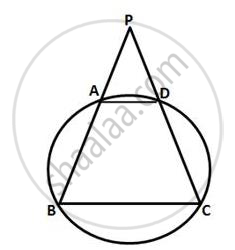Share

Books Shortlist

# Abcd is a Cyclic Quadrilateral in Which Ab and Dc on Being Produced, Meet at P Such that Pa = Pd. Prove that Ad is Parallel to Bc. - ICSE Class 10 - Mathematics

#### Question

ABCD is a cyclic quadrilateral in which AB and DC on being produced, meet at P such that PA
= PD. Prove that AD is parallel to BC.

#### SolutionLet ABCD be the given cyclic quadrilateral
Also, PA = PD (Given)

And ∠CDA = 180° - PDA = 180° - ∠PAD (From (1))
We know that the opposite angles of a cyclic quadrilateral are supplementary
∴ ∠ABC = 180° - ∠CDA = 180° - (180° - ∠PAD) = ∠PAD